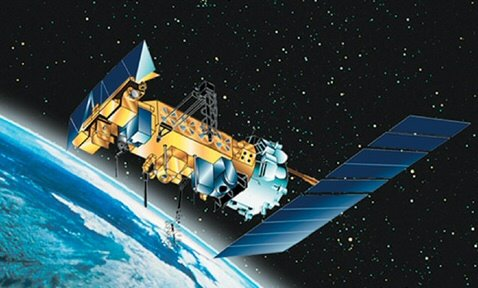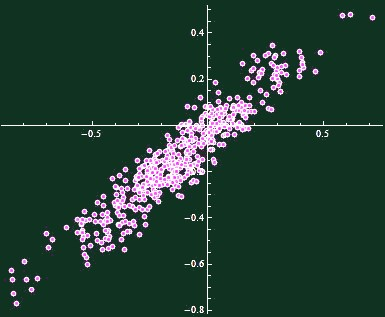## Monday, July 05, 2010 ...//### How likely it is for 2010 to be warmer than 1998

The nearly final temperature readings of UAH AMSU for June 2010 are out and the global temperature anomaly of 0.44 °C significantly - by 0.16 °C - differs from my estimates based on a different satellite which is used by the daily UAH data.So June 2010 was 0.13 °C cooler than June 1998 and it is the coolest month of 2010 so far. Also, the average anomaly of the first six months (H1) of 2010, 0.56 °C, is cooler than H1 of 1998, 0.64 °C, by 0.08 °C. Of course, if this temperature difference continued for H2 of 2010, 2010 would be cooler than 1998.

But H2 is different from H1 so this simple extrapolation isn't possible. You may ask: how likely it is for 2010 to be cooler than 1998 if we know that H1 of 2010 was 0.08 °C cooler than H1 of 1998? To answer this question statistically, let's simply look at 31 years of the UAH AMSU record, 1979-2009.

We may look at (31 choose 2) = 465 pairs of years. For each of them, we may calculate the difference of the average temperatures of their first halfs, H1, and the difference of the average annual temperatures. The H1 differences will be shown on the x-axis and the annual (H1+H2)/2 differences will appear on the y-axis of the chart below:A pretty nice, straight line with a pretty nice, normal "noisy" distribution away from the line itself. Linear regression gives

yearDifference = -0.038 + 0.82 h1Difference
Note that the slope, 0.82, is positive but smaller than one. We may say that while H2 tends to be correlated with H1, H2-H1 is actually negatively correlated with H1 itself, so H2 acts to reduce the temperature difference between two years in most cases.

Now, the individual data points deviate from the idealized line. What is the root mean square of the differences? The answer turns out to be 0.073 °C. This is the "thickness" of the line in the chart above in the y-direction.

It's enough for us to make a prediction for 2010, using the comparison with 1998.

The H1 was 0.08 °C cooler than in 1998. Multiply it by the slope, 0.82, to see that the whole year 2010 is predicted to be 0.065 °C cooler than the year 1998. At least, that's the central value. However, this predicted difference has some normally distributed noise whose standard deviation is 0.073 °C, as we said.

That means that the predicted difference is 1.12 standard deviations (0.073/0.065). Use the error function, or (approximately) this graph,to see that the probability that 2010 will still end up being warmer than 1998, according to UAH AMSU, is about 13 percent; the formula in Mathematica is (1 + Erf[-1.12/Sqrt])/2. The odds are about 6-to-1 that 2010 will stay cooler than 1998.

Using three months only

However, you may also think that the latest three months - Q2 of 2010 or April, May, June 2010 - may be better to predict the second half of the year than the whole H1 of 2010. In fact, you would be demonstrably right. If you used this algorithm and replaced H1 by Q2 in all the calculations above, the slope would become 0.81.

The root mean square would be 0.085 °C. However, the actual difference of the averages of Q2 of 1998 and Q2 of 2010 is 0.17 °C, much bigger than before. If multipled by 0.81, you get the expected difference of 1998 and 2010 means equal to 0.135 °C. That's 0.135/0.085 = 1.59 standard deviations. The corresponding probability that 2010 will still beat 1998 will come out as 5.6 percent only. The odds are 17-to-1.

Whether the odds are 6-to-1 or 17-to-1, you should better assume that 2010 will be cooler but you don't want to bet your life on it. ;-)

Bonus: No hockey stick in China

A new paper in GRL reconstructs the temperatures in various parts of China. The final charts look like this:Michael Mann is too big a communist for China for the Chinese officials to allow his flawed ideas to distort their proxies. ;-)